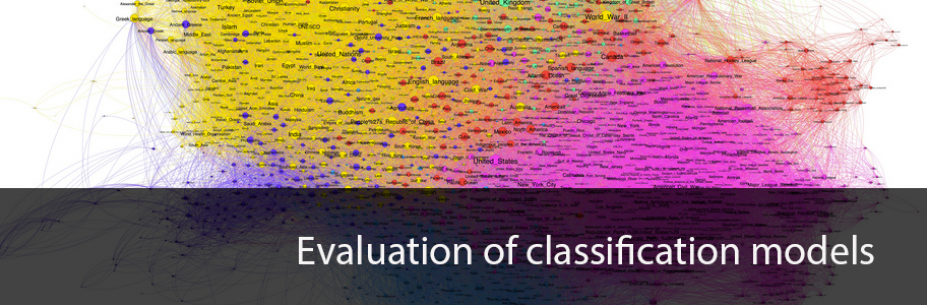# Evaluation of machine learning classification modelsGerman version of this video: https://youtu.be/rtyJyzqeByU

This is basically the content of the video for those who can't or don't want to watch the video but i would still recommend the video because there are graphical explanations of some parts of this content.

In todays video I will cover 5 measures for the quality of a classification model.
We will use the model from the last video about sentiment analysis as the model to measure.

So if you haven't watched it..although it is not must for this video...now would be a good time ;)

So let's dive in!

### Accuracy

If you watched the video about sentiment analysis you already have seen one measure technique for
classification models.

This measure is called accuracy and is calculated like:

accuracy = (right predictions) / (all samples)

or in python with scikit-learn:

```from sklearn.metrics import accuracy_score print("accuracy: " + str(accuracy_score(y_test, predicted))) ```

Our current model has an accuracy of 94%... but is it good?

### Majority class

To answer that lets have a look at the test dataset since this is the dataset with which we evaluate our model.

We have:

Total number of observations: 38909
Positives in observation: 31135
Negatives in observation: 7774
Majority class is: 80.0200467758%

As we see the data is not balanced!
There are much more positive than negative reviews in our observations.

So there is one clear majority class in this case the class "positive reviews".
This tells us that if we would simply always predict every review as positive our
accuracy would be equal the majority class.

This means that our classification model should always beat the majority class.
Else it would be better just to assume a prediction is in the majority class.

So with our accuracy of 94% we beat the majority class... which is a good thing!
Our model is better than always predicting "positive review".. yeahy!!

The next measure I would like to show is called confusion matrix

### Confusion Matrix

There is a build in method in scikit to generate a confusion matrix...

The output of this method looks like:

```# true negatives is C_{0,0}, # false negatives is C_{1,0}, # true positives is C_{1,1} and # false positives is C_{0,1}.```

```from sklearn.metrics import confusion_matrix print("confusion matrix: \n" + str(confusion_matrix(y_test, predicted)))```

confusion matrix:
[[ 6166  1608]
[  638 30497]]

I added some comments from the documentation to show you what the numbers mean...

Each prediction our model does falls in one of those four categories either it is a

true positive that means it is a positive review and also was predicted as positive

false positive that stands for a negative review that was predicted as a positive

false negative is a positive review that was predicted as negative

true negative means a negative review was correctly predicted

Depending on the problem you are working on it is possible that one of
false positives or false negatives has a higher cost... and should be considered more
harmful for the model than the other... an thus needs to be minimized... a common example for this
is when you try to predict whether someone has cancer...

In this case false negatives would mean that you tell someone he is healthy and no further treatment is needed... but in reality this is not true

A false positive in this case wouldn't be that bad... you would tell someone that he has cancer... which might be a shock... but chances are high that during the further procedure it will become clear that this is not the case and that the person is healthy..

So in the cancer prediction case you should really try to minimize false negatives!

The next measure is called precision...

### Precision

It is calculated as:

Precision = (true positives) / (true positives + false positives)

or in python with scikit-learn:

```from sklearn.metrics import precision_score print("precision: " + str(precision_score(y_test, predicted)))```

As you see from this calculation the precision tells us how precise our prediction of positive values is.
A low precision indicates many false positives.

So if false positives are causing you trouble precision is a good measure to use to fine-tune your model.

### Recall

Recall is calculated as:

Recall = (true positives) / (true positives + false negatives)

or in python with scikit-learn:

```from sklearn.metrics import recall_score print("recall: " + str(recall_score(y_test, predicted)))```

The recall tells us how many % of the observed positives were correctly predicted and therefore recall is also called
True Positive Rate.

A low recall indicates many false negatives

If false negatives are having a higher cost in the problem you try to predict, like in the cancer example from above.

### F1-Score

The F1-Score is calculated like:

F1 = 2 * (precision * recall) / (precision + recall)

or in python with scikit-learn:

```from sklearn.metrics import f1_score print("f1 score: " + str(f1_score(y_test, predicted)))```

The F1-Score combines precision and recall and is basically a weighted average of those.

### Summary

So when evaluating your classification models you shouldn't only count on accuracy but also check the other
measures. There are situations in which you really want to avoid one error more than the other..
like shown in the cancer example.